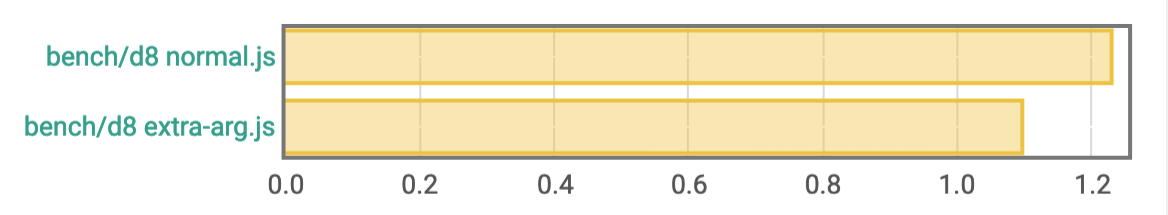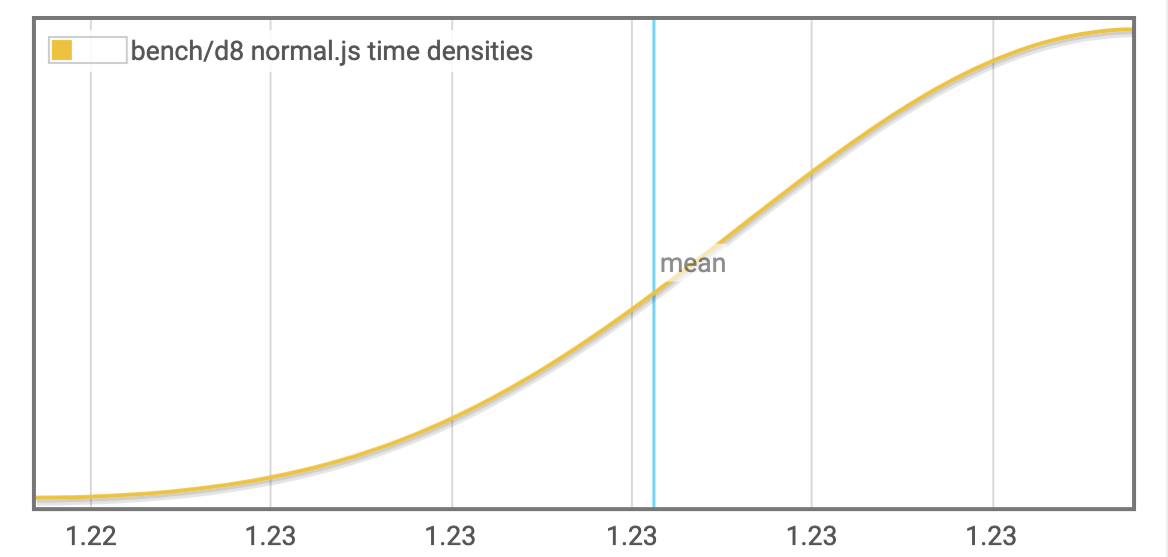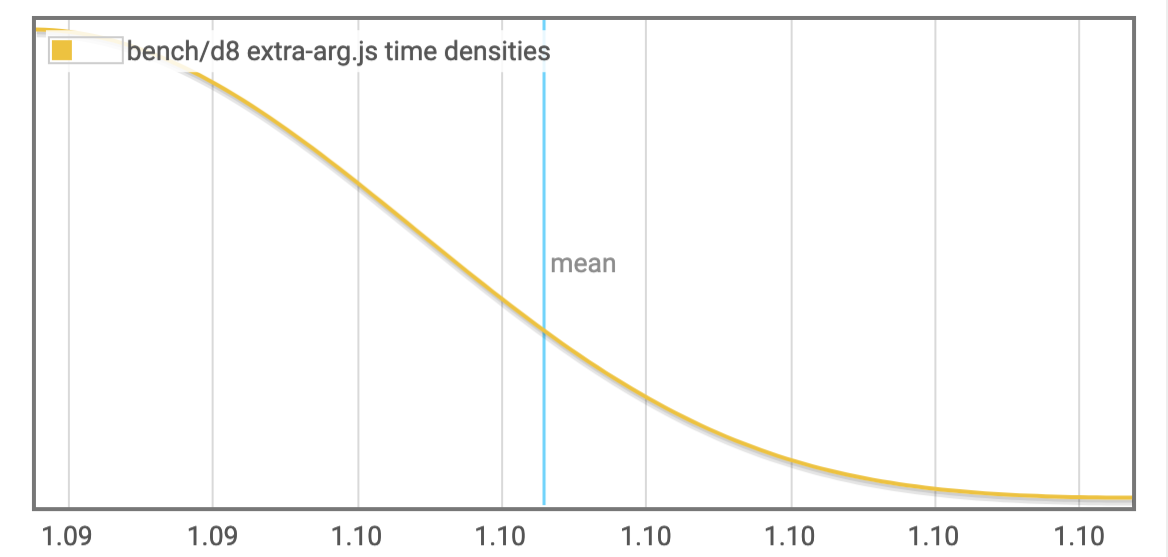Feb 11, 2017

V8 puzzle

Last week I randomly ran into a V8 behaviour I didn't expect.

Here is a Primes prototype:

function Primes() {
this.counter = 0;
this.primes = [];

this.getCounter = () => this.counter;
this.getPrime = (i) => this.primes[i];
this.push = (i) => this.primes[this.counter++] = i;
this.isPrime = (n) => {
for (var i = 1; i < this.counter; ++i) {
if ((n % this.primes[i]) == 0) return false;
}
return true;
}
}
1
2
3
4
5
6
7
8
9
10
11
12
13
14

As you can see, it doesn't take parameters. You would simply do new Primes(), not new Primes(2, ['foo', bar()], Math.random() * baz()).

Here is a main function using Primes to generate the n first prime numbers, putting them in a list:

function main(n) {
const p = new Primes();
let c = 1;
while (p.getCounter() < n) {
if (p.isPrime(c)) {
p.push(c);
}
c++;
}
print(p.getPrime(p.getCounter() - 1));
}

// and here is how I call it:
main(25000);
1
2
3
4
5
6
7
8
9
10
11
12
13
14

Now let's make two versions of this script. The only difference between the first version, normal.js, and the second version, extra-arg.js, is this one:

normal.jsfunction Primes() {
this.counter = 0;
this.primes = [];

this.getCounter = () => this.counter;
this.getPrime = (i) => this.primes[i];
this.push = (i) => this.primes[this.counter++] = i;
this.isPrime = (n) => {
for (var i = 1; i < this.counter; ++i) {
if ((n % this.primes[i]) == 0) return false;
}
return true;
}
}

function main(n) {
const p = new Primes();
let c = 1;
while (p.getCounter() < n) {
if (p.isPrime(c)) {
p.push(c);
}
c++;
}
print(p.getPrime(p.getCounter() - 1));
}

main(25000);
1
2
3
4
5
6
7
8
9
10
11
12
13
14
15
16
17
18
19
20
21
22
23
24
25
26
27
28
extra-arg.jsfunction Primes() {
this.counter = 0;
this.primes = [];

this.getCounter = () => this.counter;
this.getPrime = (i) => this.primes[i];
this.push = (i) => this.primes[this.counter++] = i;
this.isPrime = (n) => {
for (var i = 1; i < this.counter; ++i) {
if ((n % this.primes[i]) == 0) return false;
}
return true;
}
}

function main(n) {
const p = new Primes(null); /* ?! */
let c = 1;
while (p.getCounter() < n) {
if (p.isPrime(c)) {
p.push(c);
}
c++;
}
print(p.getPrime(p.getCounter() - 1));
}

main(25000);
1
2
3
4
5
6
7
8
9
10
11
12
13
14
15
16
17
18
19
20
21
22
23
24
25
26
27
28
In the *normal* version, I create an object by using `Primes` as expected: `new Primes()`. In the *extra-arg* version, I pass an extra argument like this: `new Primes(null)`. Guess what?
❯ d8
V8 version 5.8.101
❯ make
time d8 normal.js
287107
1.21 real         1.18 user         0.02 sys
time d8 extra-arg.js
287107
1.07 real         1.04 user         0.01 sys
1
2
3
4
5
6
7
8
9

The extra-arg version consistently runs ~10% faster on v8 5.8.9and 5.8.101:bench/d8 normal.jslower bound estimate upper bound
OLS regression xxx xxx xxx
R² goodness-of-fit xxx xxx xxx
Mean execution time 1.2244014127760094 1.2302440249237707 1.2336210836091757
Standard deviation 0.0 5.241324404964492e-3 5.849237223263123e-3

Outlying measurements have moderate (0.18749999999999997%) effect on estimated standard deviation.

bench/d8 extra-arg.jslower bound estimate upper bound
OLS regression xxx xxx xxx
R² goodness-of-fit xxx xxx xxx
Mean execution time 1.094407024081591 1.0972965331160693 1.0994041288091074
Standard deviation 0.0 3.19627481050371e-3 3.650462822155438e-3

Outlying measurements have moderate (0.18749999999999997%) effect on estimated standard deviation.

(Benchmark done using bench (opens new window), report generated by criterion (opens new window).)

As we can see, with 0 argument it's slow, with 1 it's fast. What happens as we keep adding more arguments? (List index is number of arguments.)

1. fast
2. slow
3. fast
4. fast
5. slow
6. fast
7. fast
8. slow
9. fast
10. slow
11. fast
12. fast
13. slow
14. fast
15. fast

My attempts at understanding what's happening here failed. I really don't know the reason behind these differences.

I ran the two original versions, the one with zero argument and the one with one argument, using d8 built with debug options:

d8 \
--trace-hydrogen \
--trace-phase=Z \
--trace-deopt \
--hydrogen-track-positions \
--redirect-code-traces \
--print-opt-code
1
2
3
4
5
6
7
8

Here are the generated files if you want to analyze them yourself:

If anyone can explain what happens here, please ping me on twitter _vhf (opens new window) or send me an email (contact@ this domain (draft.li)! I'll link to your explanation or include it here or rephrase it here.

The number of instructions fetched on a given cycle is dependent on multiple factors. For Intel's fourth generation Core processors, when using the instruction cache rather than the µop cache, an aligned 16 byte block of instructions are fetched each cycle.

https://stackoverflow.com/questions/32282452/if-the-number-of-fetched-instructions-per-cycle-is-constant-for-out-of-order-sup (opens new window)

To get a better understanding of why and how alignment matters, check out Agner Fog's the microarchitecture doc, esp. the section about the instruction-fetch front-end of various CPU designs.

https://stackoverflow.com/questions/18113995/performance-optimisations-of-x86-64-assembly-alignment-and-branch-prediction (opens new window)